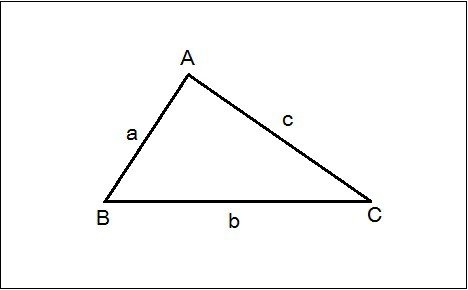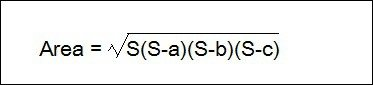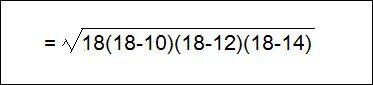# How To Calculate Land Area Or Plot Area

## How To Calculate Land Area Or Plot Area:

Sometimes we may need to know the area of any land or plot for various reasons such as buying, selling of land, or constructing house/building etc. In this article, I will explain how to calculate land are or plot area for a site.

Area Formula For Different Shapes:But for complex shaped land you cannot easily calculate the area. In that case, we use HERON’s Formula which is given below:Where S = Perimeter of the triangle

S = (a + b + c)/2

a = Distance of AB

b = Distance of BC

c =  Distance of AC

Let’s take an example,First of all, divide the land area into minimum possible no. of triangle. Then measure all the required distance (like AB, AC, AE etc) by using a tape or chain.

## 1. For △ ACD:

AC = 10 feet.

CD = 12 feet.

AD = 14 feet.

S = (a + b + c)/2 = (10 + 12 + 14)/2 = 18 feet.## 2. For △AED:

AD = 14 feet.

AE = 14feet.

DE = 12 feet.

S = (a + b + c)/2 = (14+14+12)/2 = 20 feet.## 3. For △AEB:

AB = 16 feet.

BE = 12 feet.

AE = 12 feet.

S = (a + b + c)/2 = (16 + 12 + 12)/2 = 20 feet.Total Area Of Land = 58.78 + 75.89 + 71.55 = 206.22 ft²

### Note:

This method does not give appropriate result for curve shaped area, for curve shaped area we need to use difinite integral method.